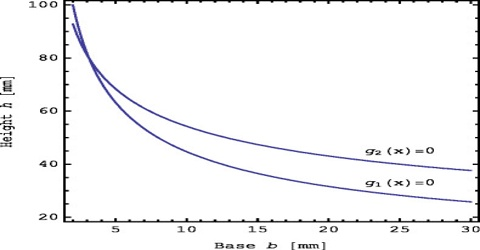# State of a Thermodynamic System and State Functions

State of a thermodynamic system and state functions (variables)

A thermodynamic system is considered to be in a definite state when each of the macroscopic properties of the system has a definite value. A state function is a property whose value does not depend on the path taken to reach that specific value. In contrast, functions that depend on the path from two values are call path functions. Both path and state functions are often encountered in thermodynamics. The state of a thermodynamic system is completely defined by four measurable macroscopic properties: pressure, volume, temperature and composition.

State functions are defined by comparing them to path functions. As stated before, a state function is a property whose value does not depend on the path taken to reach that specific function or value. In essence, if something is not a path function, it is probably a state function. To better understand state functions, first define path functions and then compare path and state functions. These properties upon which the state of a system is dependent are called stale variables or state functions. It is not necessary to know all the state functions to clearly define the state of a system. For example, if the composition of a given mass of an ideal gas is fixed, the remaining variables are related by an equation of state; PV = RT. If any two of the three variables P, T and V are known, the third is atomically calculated. In this case the two known variables are designated as independent state variable, while the third unknown variable is called dependent state variable.